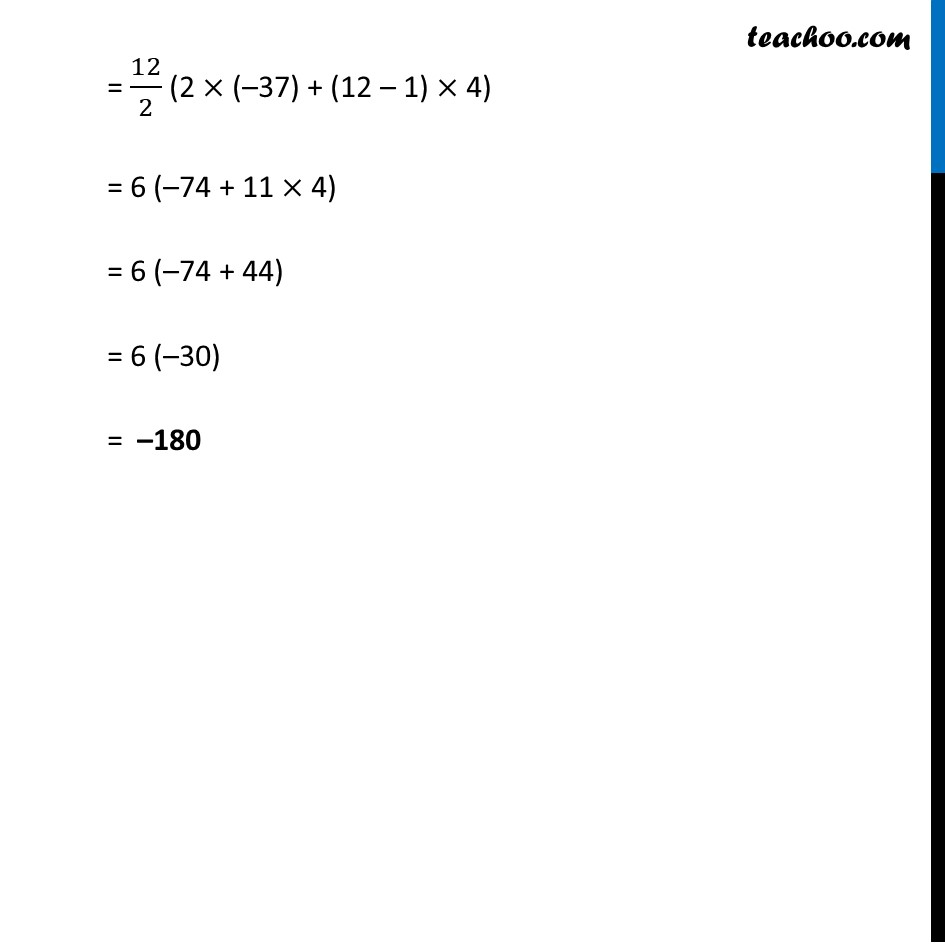1. Chapter 5 Class 10 Arithmetic Progressions (Term 2)
2. Serial order wise
3. Ex 5.3

Transcript

Ex 5.3, 1 Find the sum of the following APs. (ii) −37, −33, −29 ,…, to 12 terms –37, –33, –29 ,…, to 12 terms We know that Sum = 𝑛/2 (2a + (n – 1)d) Here n = 12, a = –37 & d = – 33 – (–37) = – 33 + 37 = 4 Putting values in formula Sum = 𝒏/𝟐 (2a + (n – 1) d) = 12/2 (2 × (–37) + (12 – 1) × 4) = 6 (–74 + 11 × 4) = 6 (–74 + 44) = 6 (–30) = –180